# What is enthalpy of reaction?

## What is enthalpy of reaction (heat of reaction) ?

1. The amount of heat given out or absorbed in a chemical reaction depends on the amount of reactants used.
2. Enthalpy of reaction or Heat of reaction is the heat change when the number of moles of reactants as shown in the chemical equation reacts in standard conditions to form products in standard conditions.
3. Standard conditions refer to the following:
(a) Temperature is 25°C or 298K
(b) Pressure is one atmospheric pressure or 101.3 kPa
(c) Concentration of solution is 1.0 mol dm-3
(d) Reactants and products are at their normal physical states of 25°C and one atmospheric pressure
4. A thermochemical equation is a balanced chemical equation that also contains its heat of reaction on the right side of the equation.

The thermochemical equation for the reaction between hydrogen and oxygen to form water is shown in the equation:
2H2(g) + O2(g) → 2H2O(l)    ∆H = – 572 kJ

• ∆H = -572 kJ is the heat of reaction. The negative sign shows that the reaction is exothermic.
• In this reaction,
(i) hydrogen and oxygen.exist in gaseous state, whereas water exists in liquid state.
(ii) 2 moles of hydrogen react with 1 mole of oxygen to form 2 moles of water.
(iii) 572 kJ of heat is produced when 2 moles of water is formed
• If the quantity of reactants is reduced to half, then the heat produced is also reduced to half as shown below.
H2(g) + 1/2 O2(g) → 2H2O(l)    ∆H = -286 kJ
• To write a thermochemical equation, fraction can be used. In the above thermochemical equation, 1 mole of hydrogen gas reacts with half a mole of oxygen to form 1 mole of water, and 286 kJ of heat is produced.

Different types of chemical reactions will give different values of heat of reaction. For a specific reaction, the heat of reaction is given a specific name as shown in Table.

 Type of reaction Heat of reaction Precipitation Heat of precipitation Displacement Heat of displacement Neutralisation Heat of neutralisation Combustion Heat of combustion

## How do you find the change in heat?

Determining heat of reaction:

1. To determine the heat of reaction in the laboratory, aqueous solution is always involved.
2. The solution is the surroundings.
3. When a reaction occurs in a solution, heat is either absorbed from the solution or given to the solution.
4. In an exothermic reaction:
Heat given out during the reaction = heat absorbed by the solution
Therefore, the temperature of the solution increases.
5. In an endothermic reaction:
Heat absorbed during the reaction = heat lost by the solution
Therefore, the temperature of the solution decreases.
6. When carrying out an experiment, we assume that no heat is lost to the surroundings. This can be achieved by conducting the experiment in a plastic cup because plastic is a heat insulator.
7. The heat change in a reaction can be calculated using the following formula:
Heat change (H) = heat absorbed or given out by the aqueous solution
H = mcθ
where m = mass of the solution (in g)
c = specific heat capacity of the solution (in J g-1 °C-1)
θ = temperature change in the solution (in °C)
8. The following are the assumptions made during the calculation of heat of reaction.
(a) The solution is dilute. It has the same density as water, which is 1 g cm-3.
(b) The solution has the same specific heat capacity as water, which is 4.2 J g-1 °C-1.
(c) No heat is lost to or absorbed from the surroundings.
(d) No heat is absorbed by the apparatus of the experiment.

## How do you calculate the enthalpy example problems with solutions

1. When 30 cm3 of potassium hydroxide solution is added to 25 cm3 of nitric acid, the results are as follows. Initial temperature of potassium hydroxide solution = 28°C
Initial temperature of nitric acid = 28°C
Highest temperature of the mixture = 38°C
Calculate the heat change in this reaction.
[Density of solution: 1 g cm-3. Specific heat capacity of solution: 4.2 J g-1 °C-1]
Solution: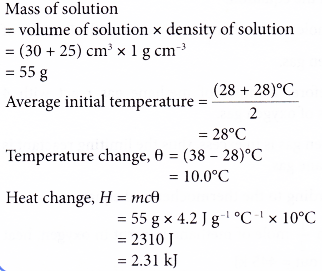2. When sulphur burns in oxygen gas, sulphur dioxide gas is produced.
S(s) + O2(g) → SO2(g)    ∆H = -297 kJ
Calculate the heat change when 20 g of sulphur is burnt completely in excess oxygen.
[Relative atomic mass: O, 16; S, 32]
Solution: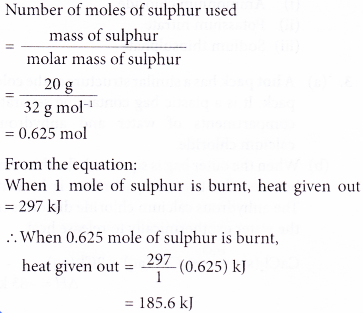3. When methane, CH4 is burnt in oxygen, water and carbon dioxide are produced. The thermochemical equation is shown below.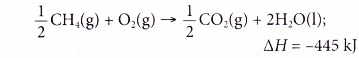How much heat is produced when 2 moles of methane are burnt in 5 moles of oxygen?
Solution: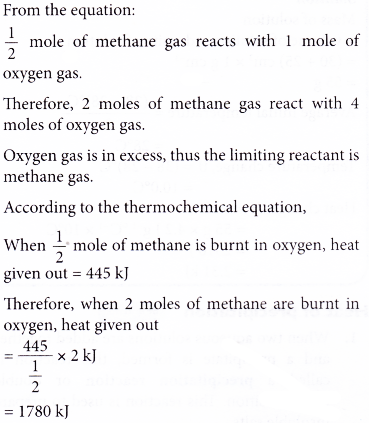4. The thermochemical equation for the reaction between nitrogen and hydrogen is as shown below.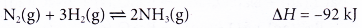What is the change in heat if 4 moles of ammonia are produced?
Solution:5. The thermochemical equation for the combustion of octane is as shown below.Calculate the mass of octane required for combustion to produce 1378 kJ of heat.
[Relative atomic mass: H, 1; C, 12]
Solution: